# Free Vibrations of a Simply-supported Thin Square PlateThis test lets you check analysis results for a simply-supported thin square plate, in the context of a free frequency case. You will use 2D meshes.

This test proposed by NAFEMS is used to validate the following attributes:

• 2D shell elements (i.e. the elementary stiffness and mass matrices)
• Free frequency solve algorithms (mainly repeated eigen values)
• Convergence of 2D shell elements (via several meshes).Reference:

NAFEMS-Glasgow, BENCHMARK newsletter, Report No. E1261/R002, February 1989, p.21.

##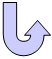Specifications

### Geometry Specifications

 Length: L = 10000 mm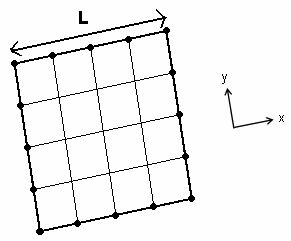Thickness: th = 50 mm

### Analysis Specifications

 Young Modulus (material): E = 200 GPa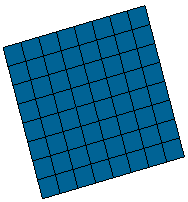Poisson's Ratio (material): ν = 0.3 Density: ρ = 8000 kg/m3 Restraints (User-defined): Tx = Ty = Rz = 0 at all nodes Tz = 0 along all four edges Rx = 0 along edges x = 0 and x = 10000 mm Ry = 0 along edges y = 0 and y = 10000 mm

##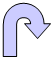Results

You will find here the results for different finite elements:

### Parabolic Triangle Shell (32 x 32 elements)

 Mode Reference frequency [Hz] Parabolic triangle shell (32 x 32 elements)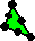Frequency [Hz] Error [%] 1 2.377 2.377 0.013 2 5.942 5.943 0.019 3 5.942 5.943 0.021 4 9.507 9.516 0.095 5 11.884 11.882 0.013 6 11.884 11.883 0.010 7 15.449 15.467 0.118 8 15.449 15.469 0.126

To visualize the convergence of parabolic triangle shell elements with other mesh refinements, click here.

### Linear Triangle Shell (64 x 64 elements)

 Mode Reference frequency [Hz] Linear triangle shell (64 x 64 elements)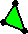Frequency [Hz] Error [%] 1 2.377 2.378 0.022 2 5.942 5.947 0.082 3 5.942 5.947 0.084 4 9.507 9.520 0.133 5 11.884 11.904 0.167 6 11.884 11.904 0.171 7 15.449 15.483 0.218 8 15.449 15.483 0.221

To visualize the convergence of linear triangle shell elements with other mesh refinements, click here.

### Parabolic Quadrangle Shell (16 x 16 elements)

 Mode Reference frequency [Hz] Parabolic quadrangle shell (16 x 16 elements)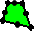Frequency [Hz] Error [%] 1 2.377 2.376 0.022 2 5.942 5.940 0.026 3 5.942 5.940 0.026 4 9.507 9.503 0.038 5 11.884 11.880 0.034 6 11.884 11.880 0.034 7 15.449 15.442 0.049 8 15.449 15.442 0.049

To visualize the convergence of parabolic quadrangle shell elements with other mesh refinements, click here.

### Linear Quadrangle Shell (32 x 32 elements)

 Mode Reference frequency [Hz] Linear quadrangle shell (32 x 32 elements)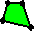Frequency [Hz] Error [%] 1 2.377 2.378 0.030 2 5.942 5.954 0.203 3 5.942 5.954 0.203 4 9.507 9.523 0.171 5 11.884 11.952 0.568 6 11.884 11.952 0.568 7 15.449 15.510 0.392 8 15.449 15.510 0.392

To visualize the convergence of linear quadrangle shell elements with other mesh refinements, click here.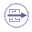To Perform the Test:

The Free_vibrations_simply_Thin_Square_Plate.CATAnalysis document presents a complete analysis of this case, computed with a mesh formed of 16 elements per edge, and parabolic quadrangle elements (QD8).

To compute the case with other mesh sizes and element types, proceed as follow:

1. Open the CATAnalysis document.

2. In the Advanced Meshing Tools workbench, replace the mesh size and element type.

3. In the Generative Structural Analysis workbench, compute the case, and activate the image called Deformed mesh.